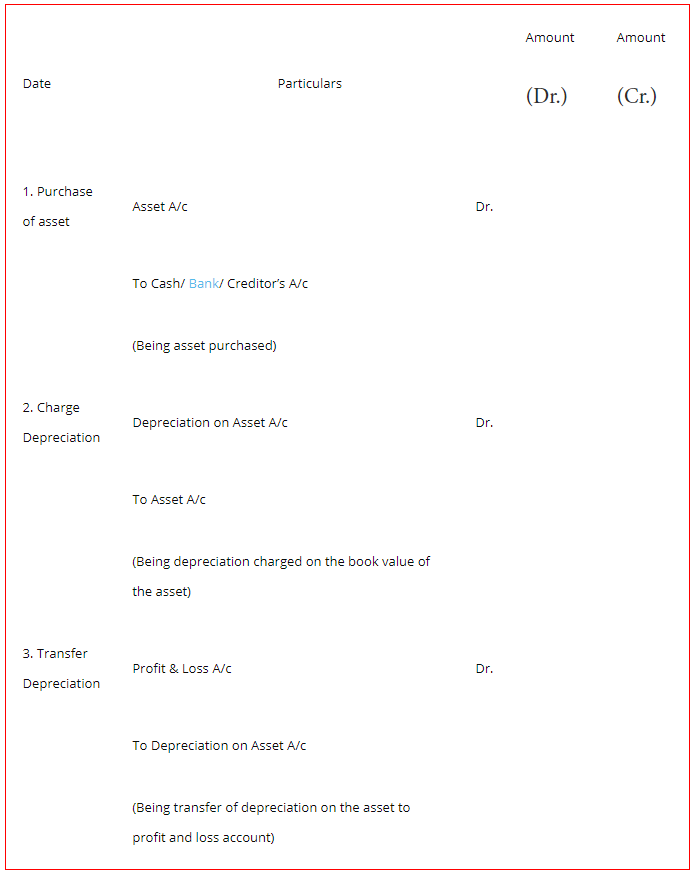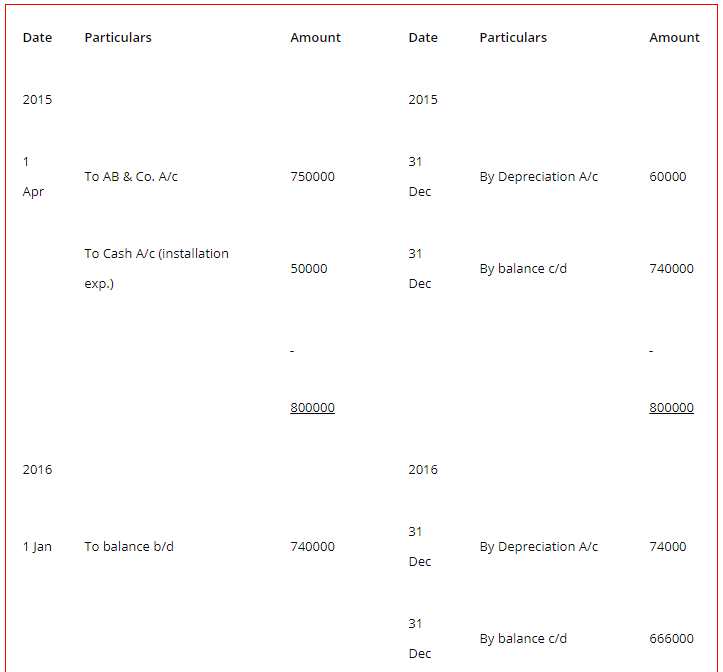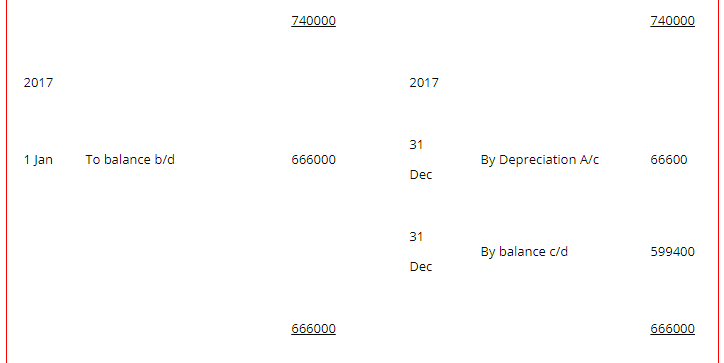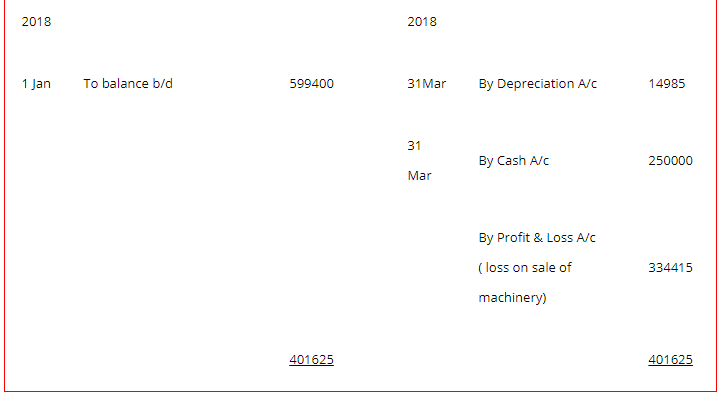# Charging Depreciation: Declining Charge Method – Examples and Types

The compilation of these Depreciation, Provision and Reserves Notes makes students exam preparation simpler and organised.

## Declining Charge Method

Depreciation is the decrease in the value of fixed assets due to normal wear and tear, efflux of time or obsolescence due to technology. Thus, depreciation is an expense and we charge it to Profit and Loss A/c at the end of the year. One of such methods of charging depreciation is the Declining Charge Method. This formula is derived from the study of the behaviour of the assets over a period of time.

### Charging Depreciation – Declining Charge Method

Under these methods, the depreciation amount decreases for every subsequent year. Usually, we apply these methods when the receipts from the assets decline and it becomes essential to charge the depreciation as per the asset’s expected earnings.

The methods of charging depreciation that fall under this category are:

1. Diminishing Balance Method
2. Sum of Years’ Digit Method
3. Double Declining Method

Diminishing Balance Method
According to the Diminishing Balance Method, we charge depreciation at a fixed percentage on the book value of the asset appearing in the Balance Sheet.

As the book value of the depreciable asset reduces every year, it is also known as the Reducing Balance Method or Written-down Value Method.

As the book value of the asset reduces every year applying this method, hence the amount of depreciation expense also reduces every year. Thus, under this method, the value of the asset never reduces to zero or becomes nil.

The assumption underlying is that in the earlier years the cost of repairs to the assets is low and hence there is a requirement to charge more amount of depreciation.

Also, in the later years, the cost of repairs will increase, and therefore the charge of depreciation will be less. Hence, as a result, there is an equal burden on the profit every year during the useful life of the asset.

This method also considers the period of use of the asset. When an asset is in use only for 4 months in a year then we charge depreciation only for 4 months.

However, the Income Tax Act states that if an asset is in use for more than 180 days, we need to charge full years’ depreciation. Income Tax Rules allow depreciation charged on the Reducing Balance Method.

Formula:
1. Rate of Depreciation = $$[1-\sqrt[n]{s / c}] \times 100$$
Where, n = useful life
s = scrap value
c = cost of asset

2. Amount of depreciation = Book Value × $$\frac{\text { RateofDepreciation }}{100}$$
Journal entry for Diminishing Balance method of Depreciation:1. This method lays an equal burden on the profit of every year as depreciation decreases every year and repair expenses increase every year.
2. It is permissible to charge depreciation using this method as per the Income Tax Act.
3. As the major part of depreciation is charged in the earlier years the problem of obsolescence is given due care. Thus, it is easy to replace the asset.

1. Under this method, it is difficult to calculate the appropriate rate of charging depreciation. Thus, it may happen that at the end of the useful life of the asset full depreciation is not provided.
2. The cost of the asset never becomes nil.
3. This method does not distribute the depreciation uniformly throughout the useful life of the asset.

Example:

Question:
M/s. Bhanu and his sons purchase a machine on 1 Apr 2015 for ₹750000 from AB & Co. and paid ₹50000 on its installation. The estimated useful life of the machine is 3 years. The estimated residual value of the machine is ₹40000. On 31st March 2018, the machine was sold for 250000. Depreciation is charged as per the W.D.V. method @10 % p. a. Prepare the necessary ledger accounts for the year ending 31st December every year for charging depreciation.
In the books of M/s. Bharat and sons
Machinery A/cDepreciation A/cWorking Notes:
Calculation of the amount of depreciation
Depreciation = Book Value × $$\frac{Rateofdepreciation}{100}$$

2015
Depreciation = 800000 × $$\frac{10}{100} \times \frac{9}{12}$$ = 60000

2016
Depreciation = 740000 × $$\frac{10}{100}$$ = 74000

2017
Depreciation = 666000 × $$\frac{10}{100}$$ = 66600

2018
Depreciation = 599400 × $$\frac{10}{100} \times \frac{3}{12}$$ = 14985

2. Calculation of loss on sale of machinery
Loss = Book Value on 1st Jan 2018 – depreciation for 3 months – Cash received on the sale
= 599400 – 14985 – 250000
= 334415